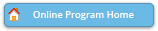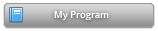#### Abstract Details

 Activity Number: 441 - Recent Advances in Nonparametric Statistics Type: Invited Date/Time: Wednesday, July 31, 2019 : 8:30 AM to 10:20 AM Sponsor: IMS Abstract #300359 Title: ISOTONIC REGRESSION in MULTI-DIMENSIONAL SPACES and GRAPHS Author(s): Hang Deng* and Cun-Hui Zhang Companies: Rutgers University and Rutgers University Keywords: isotonic regression; minimax rate; block estimator; adaptive estimation; variable selection; multiple isotonic regression Abstract: We study minimax and adaptation rates in general isotonic regression. The LSE is known to be identical on every design point to both the max-min and min-max estimators over all upper and lower sets containing the design point. We however consider the block estimators which lie in-between the max-min and min-max estimators over hyper-rectangular blocks. Under a q-th moment condition on the noise, we develop \ell_q risk bounds for such general estimators for isotonic regression on graphs. For uniform deterministic and random designs in [0,1]^d with d>2, our mean squared risk bound for the block estimator matches the minimax rate n^{-1/d} and achieves the near parametric adaptation rate (K/n)\{log(n/K)\}^d when the unknown mean function is piecewise constant on K hyper-rectangles. More interestingly, the block estimator possesses the oracle property in variable selection: When the unknown mean function depends on only a subset of S variables, the mean squared risk of the block estimator automatically achieves minimax rate for known S. We show the block estimators enjoy tighter risk bounds in terms of the above three types of rates.

Authors who are presenting talks have a * after their name.